• 1.如何保证三维图像的维度和图像维度一致 2.怎么把彩色RGB图像放上去c++ c++
• 本代码主要利用MATLAB工具实现MATLAB——设置figure、axes和surface函数属性，简单明了，易于理解
• 可以看出，MATLAB基本的三维图形包括线型(line)、网格型(mesh)、区域型(area)、面型(surface)、方向矢量型(direction)、容积型(volumetric)等多种类型，图中已经将各个函数所能够绘制图形的基本样式做了小的缩略图。...


7.3  三维图形除了绘制二维图形，MATLAB还提供了一系列强大的三维图形绘制函数，这些函数的分类列表如图7-29所示。可以看出，MATLAB基本的三维图形包括线型(line)、网格型(mesh)、区域型(area)、面型(surface)、方向矢量型(direction)、容积型(volumetric)等多种类型，图中已经将各个函数所能够绘制图形的基本样式做了小的缩略图。本节介绍常用三维绘图函数的使用。至于其他的绘图函数，因篇幅有限，这里不再介绍，请读者查阅帮助文档。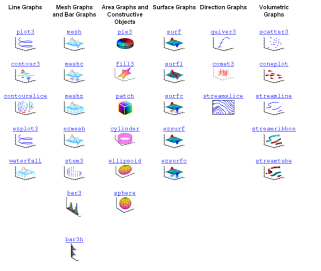图7-29  三维图形绘制函数分类列表7.3.1  绘制三维曲线图在MATLAB中，plot3函数用于绘制三维曲线图。该函数的用法和plot类似，其调用语法如下。(1)plot3(X1,Y1,Z1,...)： X1、Y1、Z1为向量或者矩阵。当X1、Y1、Z1为长度相同的向量时，此函数将绘制一条分别以向量X1、Y1、Z1为x、y、z坐标的空间曲线；当X1、Y1、Z1为矩阵时，该命令以每个矩阵的对应列为x、y、z坐标绘制出m条空间曲线。(2)plot3(X1,Y1,Z1,LineSpec,...) ：通过LineSpec设置曲线和点的属性。(3)plot3(...,'PropertyName',PropertyValue,...)：利用指定的属性绘制图形。(4)h = plot3(...)：返回一个图形对象句柄的列向量。【例7-26】  绘制三维螺旋线。Ex_7_26.mt = 0:pi/50:10*pi;plot3(sin(t),cos(t),t)grid onaxis square以上代码运行的结果如图7-30所示。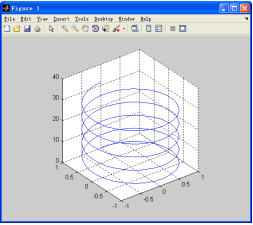图7-30  三维螺旋线7.3.2  绘制三维曲面图在MATLAB中，除了plot3函数可用于绘制三维图形外，还有一些函数可以用来绘制三维网格图和曲面图。下面分别介绍这些函数。1．三维网格图mesh函数用于绘制三维网格图，其调用语法如下。(1)mesh(X,Y,Z)：绘制出一个网格图，图像的高度由Z来决定，另外图像的颜色也由Z确定，即图像的颜色与高度成正比。如果X和Y为向量，那么length(X)= n，且(2)ength(Y) = m，其中[m,n]= size(Z)，在绘制的图形中，网格线上的点由坐标(X(j), Y(i), Z(i,j))决定。向量X对应于矩阵Z的列，向量Y对应矩阵Z的行。(3)mesh(Z)：以Z的元素为z坐标，元素对应矩阵的行数和列数分别为x和y坐标。(4)mesh(...,C)：C为矩阵。绘制出的图像的颜色由C指定。MATLAB对C进行线性变换，得到颜色映射表。如果X、Y、Z为矩阵，矩阵的维数则应该与C相同。(5)mesh(...,'PropertyName',PropertyValue,...)：利用指定的属性绘制图形。(6)mesh(axes_handles,...)：利用指定的坐标轴绘制，axes_handles为坐标轴句柄。(7)meshc(...)：创建一个匹配有二维等高线图的网格图。(8)meshz(...)：绘制出网格周围的参考面。(9)h = mesh(...)：返回一个图形对象的句柄。【例7-27】  绘制函数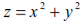的网格图。Ex_7_27.mx=-4:.2:4;y=x;[X,Y]=meshgrid(x,y); Z=X.^2+Y.^2;mesh(X,Y,Z)以上代码运行的结果如图7-31所示。【例7-28】  绘制peaks函数的三维网格图及其在底面投影的等高线图。Ex_7_28.m[X,Y] = meshgrid(-3:.125:3);Z = peaks(X,Y);meshc(X,Y,Z);axis([-3 3 -3 3 -10 5])以上代码运行的结果如图7-32所示。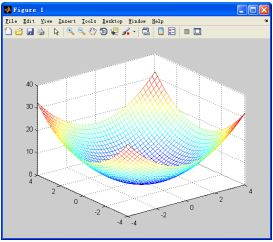图7-31  三维网格图图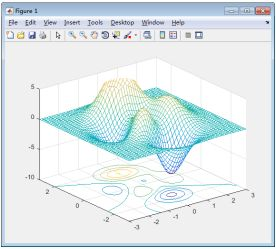7-32  peaks函数三维网格图及其在底面投影的等高线图2．三维曲面图函数surf用来绘制三维表面图形，其调用语法如下。(1)surf(Z)和surf(Z,C)：在这两个用法中，X默认为X=1:n，Y默认为Y=1:m，此时Z是一个单值函数。(2)surf(X,Y,Z)：如果X和Y为向量，那么length(X)= n，且length(Y) = m，其中[m,n]= size(Z)，在绘制的图形中，网格线上的点由坐标(X(j), Y(i), Z(i,j))决定。向量X对应矩阵Z的列，向量Y对应矩阵Z的行。(3)surf(X,Y,Z,C)：通过4个矩阵参数绘制彩色的三维表面图形。其中，图形的视角由view函数值定义；图形的各轴范围由X、Y、Z通过当前的axis函数值定义；图形的颜色范围由C定义。(4)surf(...‘PropertyName’,PropertyValue,...)：设置图形表面的属性值，单个语句可以设定多个属性值。(5)surf(axes_handles,...)：利用指定的坐标轴绘制，axes_handles为坐标轴句柄。(6)surfc(...)：创建一个匹配有二维等高线图的曲面图。(7)h = surf(...)：返回一个图形对象的句柄。【例7-29】  绘制peaks函数的曲面图。Ex_7_29.m[X,Y,Z] = peaks(30);surfc(X,Y,Z)colormap hsvaxis([-3 3 -3 3 -10 10])以上代码运行的结果如图7-33所示。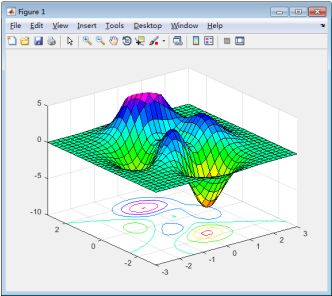图7-33  peaks函数曲面图7.3.3  特殊三维图形1．三维条形图在MATLAB中，可以使用函数bar3和bar3h来绘制三维条形，它们的调用语法与前面讲的函数bar和barh相似，这里不再赘述。【例7-30】  使用bar3和bar3h函数绘制条形图示例。Ex_7_30.mX=rand(5,5)*10;  %  产生5×5矩阵，其中每个元素为1~10之间的随机数subplot(221),bar3(X,'detached'),title('detached');subplot(222),bar3(X,'grouped'),title('grouped');subplot(223),bar3h(X,'stacked'),title('stacked');subplot(224),bar3h(X,'detached'),title('detached');以上代码运行的结果如图7-34所示。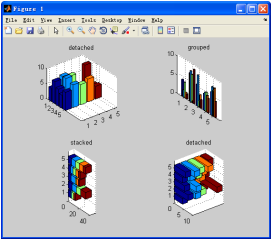图7-34  三维条形图示例2．三维球体图MATLAB提供了sphere函数来生成三维球体图。【例7-31】  sphere函数使用示例。Ex_7_31.msubplot(2,2,1)sphere(8)            %  括号中的数字指生成球体的面数，这里是指8×8axis equalsubplot(2,2,2)sphere(16)axis equalsubplot(2,2,3)sphere(24)axis equalsubplot(2,2,4)sphere(32)axis equal以上代码运行的结果如图7-35所示。3．三维饼形图函数pie3用于绘制三维饼形图，其用法与二维饼形图函数pie基本相同。【例7-32】  使用函数pie3绘制三维饼形图。Ex_7_32.mx=rand(1,5);               %  产生一个含有5个0~1之间的随机数构成的向量explode=[0 1 0 0 0];      %  分离出向量x的第二个元素pie3(x,explode)以上代码运行的结果如图7-36所示。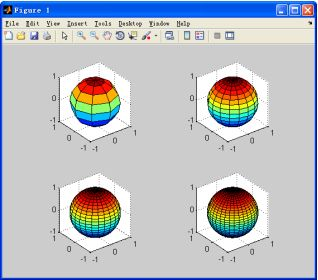图7-35  三维球体图示例图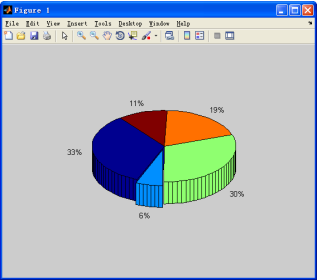7-36  三维饼形图示例4．三维箭状图函数quiver3用来绘制三维的箭状图或速度矢量图，其用法和quiver类似。【例7-33】  绘制曲面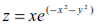的曲面法线。Ex_7_33.m[X,Y] = meshgrid(-2:0.25:2,-1:0.2:1);Z = X.* exp(-X.^2 - Y.^2);[U,V,W] = surfnorm(X,Y,Z);quiver3(X,Y,Z,U,V,W,0.5);hold onsurf(X,Y,Z);colormap hsvview(-35,45)axis ([-2 2 -1 1 -.6 .6])hold off以上代码运行的结果如图7-37所示。5．三维等高线图contour3函数用于绘制一个矩阵的三维等高线图，其用法与contour函数基本相同。【例7-34】  绘制函数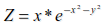的等高线图形，并使用cool颜色图。Ex_7_34.m[X,Y] = meshgrid([-2:.25:2]);   %  生成维数相同的两个矩阵X，YZ = X.*exp(-X.^2-Y.^2);contour3(X,Y,Z,40)           %  绘制Z的等高线，40为等高线的数目surface(X,Y,Z,'EdgeColor',[.8 .8 .8],'FaceColor','none')%  绘制表面图grid off                     %  去掉网格线view(-15,25)                %  设定视角colormap cool               %  建立颜色图以上代码运行的结果如图7-38所示。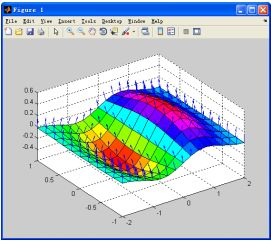图7-37  曲面法线图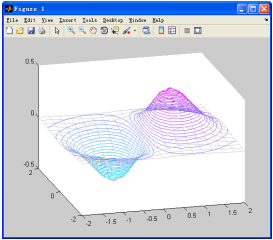图7-38  三维等高线图示例展开全文• 1. surf 绘图函数 surf 是 surface 的缩写，表示表面（显然至少三维图像才会有表面）；
plot：画线（curve，二维空间以及三维空间）
surf：画面（surface，一般在三维空间）
1. surf 绘图函数

surf 是 surface 的缩写，表示表面（显然至少三维图像才会有表面）；
z=3x2+3y2+3xy+1−3x−3y<!--//--><![CDATA[//><!--
z = 3x^2+3y^2+3xy+1-3x-3y
//--><!]]>自变量区域的指定

比如这样的一个定义域，D={(x,y)|x≥0,y≥0,x+y≤1}<!--//--><![CDATA[//><!--
D=\{(x,y)|x\geq 0,y\geq 0,x+y\leq 1\}
//--><!]]>

[x, y] = meshgrid(0:0.01:1);
% 步长可以适当地增大，
idx = x+y > 1;
x(idx) = NaN; y(idx) = NaN;
z = 3*x.^2+3*y.^2+3*x.*y+1-3*x-3*y;
surf(x, y, z)
% 增加显示 colorbar, 颜色与高度的对应关系；

注意颜色表示高度（也即是 z<!--//--><![CDATA[//><!--
z
//--><!]]> 的值），颜色越蓝，高度越低，越红，则越高；

2.
展开全文• 假设要绘制一个曲面函数，自变量名x,y，因变量名surface_equ，均为符号表达式。 syms x y surface_equ = -1./sqrt(x.^2+y.^2+0.1); axis_range = [-2:0.01:2]; [X,Y]=meshgrid(axis_range, axis_range); 接...
假设要绘制一个曲面函数，自变量名x,y，因变量名surface_equ，均为符号表达式。

syms x y

surface_equ = -1./sqrt(x.^2+y.^2+0.1);

axis_range = [-2:0.01:2];

[X,Y]=meshgrid(axis_range, axis_range);

接下来要把数值矩阵X，Y分别代入符号变量x，y，以求得曲面函数在第三维度Z轴上的取值。此时有两种方法：

1. 传统double(subs())方法。

Z=double(subs(surface_equ,{x,y},{X,Y}));

2. matlabFunction()方法。

mf = matlabFunction(surface_equ);

Z=mf(X,Y);

在我的七代i5，matlabR2019b上，第一种方法耗时53.646743秒，第二种0.003489秒，相差15000倍。

每个元素的结果误差在1e-15数量级。

展开全文• axis tight%Set the axis limits to equal the range of the dataaxis squareaxis 'auto x'%x轴坐标上下限自动调整axis off%Plot a surface without displaying the axes lines and background.set(gca,'Visible','...
简介peaks;axis tight%Set the axis limits to equal the range of the dataaxis squareaxis 'auto x'%x轴坐标上下限自动调整axis off%Plot a surface without displaying the axes lines and background.set(gca,'Visible','off'); %消除坐标轴，显示范围的大小没有改变，同上句tmp = gca;tmp.XAxis.Visible = 'off'; %隐藏X轴的%上面一句也可以隐藏Ticks，同时Box的上端也隐藏了，这就使得图像看上去不太好看tmp.XTick= []; %这一句可以只隐藏Ticks%更多特性可参考Matlab帮助文档，查找"Axes Properties"%例一：同时设置subplot的多幅图像的axis% Create a figure with two subplots.set the axis limits for the subplots to the same values.x1 = linspace(0,10,100);y1 = sin(x1);ax1 = subplot(2,1,1);plot(ax1,x1,y1)%x2 = linspace(0,5,100);y2 = sin(x2);ax2 = subplot(2,1,2);plot(ax2,x2,y2);%axis([ax1 ax2],[0 10 -1 1])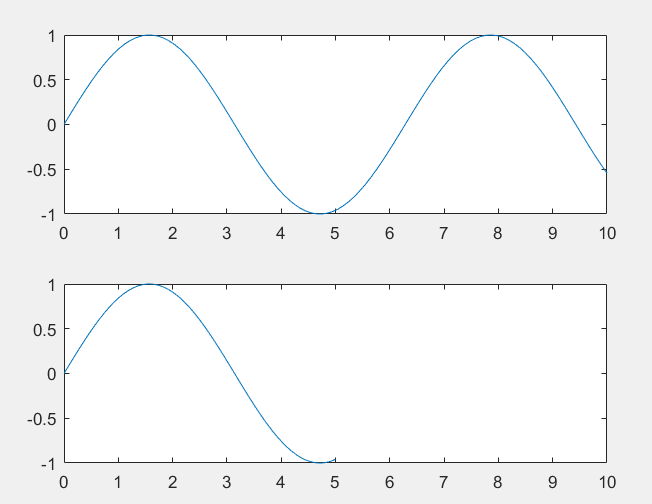%例二：在原图上继续作图，而不改变原坐标系的区间x = linspace(0,10);y = sin(x);plot(x,y)y2 = 2*sin(x);hold onaxis manual%关键步骤，冻结axis可以对比不加该语句的结果plot(x,y2);hold off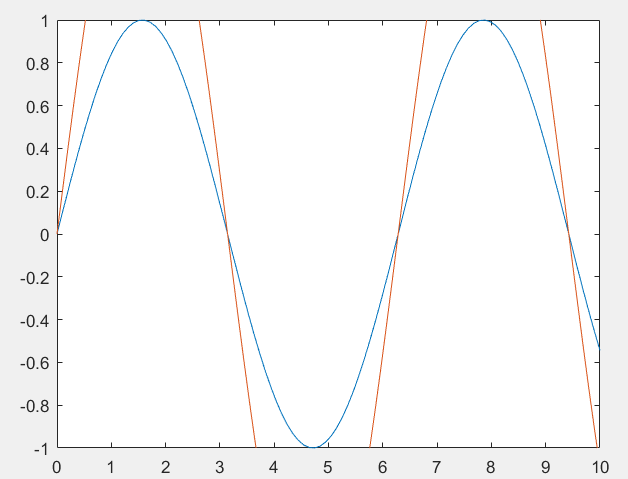%例三：改变坐标系的方向(指向)C = eye(10); pcolor(C);colormap summer% Reverse the coordinate system so that the y values increase from top to bottom.axis ij;   %第i行，第j列%上下两条语句等价set(gca,'Ydir','reverse');% y轴默认是指向上的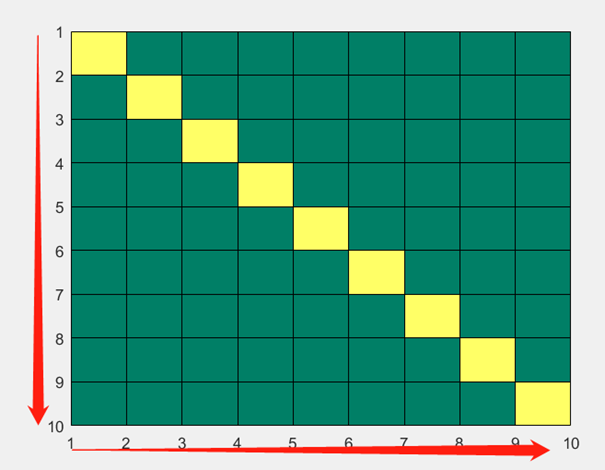实际应用：结合axis,axes,colorbar等工具，制作一个数字图像灰度统计图。%数据生成及展示(真实情况可以用数字图像代替)set(groot,'defaultAxesLineStyleOrder','remove','defaultAxesColorOrder','remove');%每次使用记得清除上次设置的参数，否则设置的参数会被保留下来x=1:255;y=rand(1,255);y=y';%y是行向量还是列向量都无所谓n=length(x);stem(x,y, 'Marker', 'none');title('未设置坐标轴的区间','fontsize',14);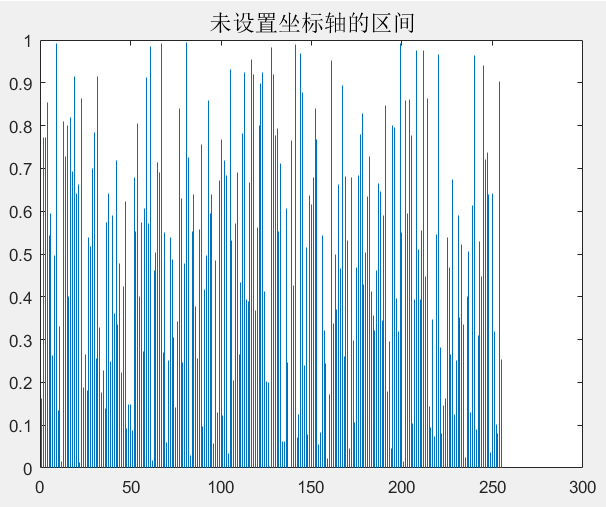%坐标轴区间的自动设置(适用于直方图的显示)% Get x/y limits of axes using axishist_axes = gca;limits = axis(hist_axes);ifn ~= 1  %当只有一个值时设置x坐标轴limits(1) = min(x);elselimits(1) = 0;endlimits(2) = max(x);var = sqrt(y'*y/length(y));limits(4) = 2*var;   %只改变了y轴显示的高度axis(hist_axes,limits);title('设置了的坐标轴区间','fontsize',14);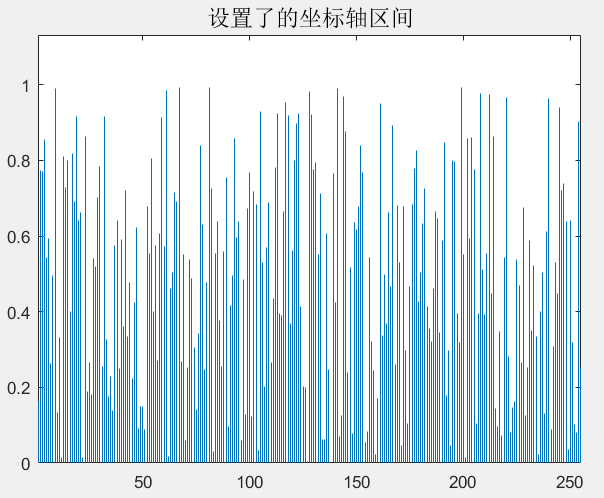%改变图像的位置，宽和高，隐藏X轴的标注% In GUIDE, default axes units are characters. In order for axes repositiong% to behave properly, units need to be normalized.hist_axes_units_old = get(hist_axes,'units');set(hist_axes,'Units','Normalized');%隐藏X轴的标注% hist_axes.XAxis.Visible = 'off';%上面一句也可以隐藏Ticks，同时Box的上端也隐藏了，这就使得图像看上去不太好看%下面这一句可以只隐藏Ticks;hist_axes.XTick= [];% Get axis position and make room for others.pos = get(hist_axes,'pos');set(hist_axes,'pos',[pos(1) 0.15 pos(3) 0.75])set(hist_axes,'Units',hist_axes_units_old);  %坐标向上移动了，相应也调整了整个图众向比例title('移动了的坐标原点','fontsize',14);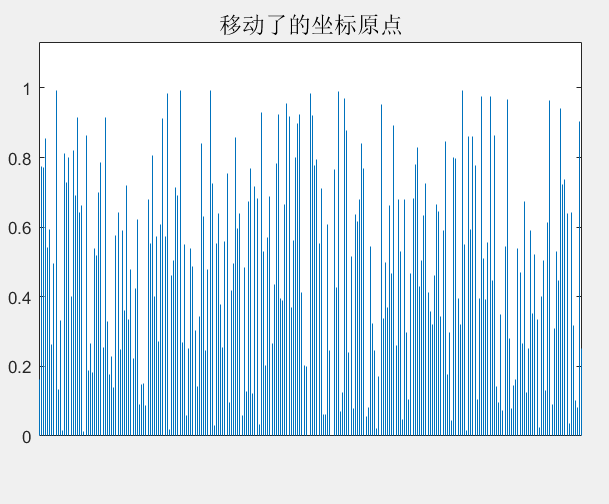%设置ColorBarc = colorbar('position',[pos(1) 0.1 pos(3) 0.05], 'location','southoutside');c.Ticks= 0:0.125:1;c.TickLabels = ceil((0:0.125:1)*255);  %使用floor函数，Ticks与真实值似乎有偏差c.Box = 'off';         %取消显示ColorBar的框框，这样使ColorBar的上框线看起来不粗c.TickDirection = 'both';colormap gray;% colorbar最左下角点的横坐标、纵坐标、宽度、高度title('一个灰度统计图的绘制就完成了','fontsize',14);展开全文• ## MATLAB中函数mesh()用法

万次阅读 多人点赞 2013-03-06 09:42:09
网格图是作为视点由view（3）设定的surface图形对象。曲面的颜色与背景颜色相同（当要动画显示不透明曲面时，这时可用命令hidden控制），或者当画一个标准的可透视的网线图时，曲面的颜色就没有（命令shading控制...
• ## Matlab中legend函数

千次阅读 2014-06-03 17:15:16
Matlab中legend函数功能    在图形上添加图例。该命令对有多种图形对象类型（线条图，条形图，饼形图等）的窗口中显示一个图例。对于每一线条，图例会在用户给定的文字标签旁显示线条的线型，标记符号和颜色等。当...
• Surface plot with colormap-based lighting Syntax surfl(Z) surfl(…,‘light’) surfl(…,s) surfl(X,Y,Z,s,k) h = surfl(…) Description The surfl function displays a shaded surface based on a combinatio....
• 当所画的是区域（patch或surface对象）时，图例会在文字旁显示表面颜色。Matlab在一个坐标轴中仅仅显示一个图例。图例的位置有几个因素决定，像遮挡的对象等，用户可以用鼠标拖动图例到恰当的位置，双击标签可以进入...
• Matlab 线性拟合 & 非线性拟合使用Matlab进行拟合是图像处理中线条...关键函数：fittypeFit type for curve and surface fittingSyntaxffun = fittype(libname)ffun = fittype(expr)ffun = fittype({expr1,…...
• ## MATLABGUI设计

千次阅读 2019-08-16 17:12:54
MATLAB提供函数用于获取图形对象的句柄，如figure、line、text、surface、axes(xlabel、ylabel、zlabel、title)等。 1、若要获取当前图形、坐标轴和对象的句柄值，可使用如下函数： gcf 获取当前图形窗口的句柄值 ...
• 这就需要Matlab的数据可视化功能，关键函数surface， light和membrane。 surface有两种用法， SURFACE(X,Y,Z,C)与SURFACE(X,Y,Z)，L = 40*membrane(1,25); logoFig = figure('Color',[0 0 0]); logoax = axes('Ca
• 可以看出，MATLAB基本的三维图形包括线型(line)、网格型(mesh)、区域型(area)、面型(surface)、方向矢量型(direction)、容积型(volumetric)等多种类型，图中已经将各个函数所能够绘制图形的基本样式做了小的缩略图。...
• ## MATLAB图形图像处理

热门讨论 2011-01-03 12:20:11
14.3.5 图像复原的其它 MATLAB 函数 第十五章 图像分析 15.1 边缘检测 15.1.1 微分算子 15.1.2 Log算子 15.1.3 Canny 算子 15.2 四叉树分解 15.2.1 四叉树分解 15.2.2 四叉树 MATLAB 函数 第十六章 数学...
• ## Matlab 线性拟合 & 非线性拟合

万次阅读 多人点赞 2012-06-22 21:24:50
使用Matlab进行拟合是图像处理中线条变换的一个重点内容，本文将详解Matlab中的直线拟合和曲线拟合用法。 关键函数： fittype Fit type for curve and surface fitting Syntax ffun = fittype(libname) ...plot random c 图像处理
• matlab中我么可以调用mesh(x,y,z)函数来产生三维图像。首先，我们用z=cos(x)sin(y)在-2pi ≤x,y≤ 2pi内的图像来看看:[x,y] = meshgrid(-2*pi:0.1:2*pi);z = cos(x).*sin(y);mesh(x,y,z),xlabel('x'),ylabel('y'),...
• 可用于将一个二维数据按区间数分割后计算每个方形区间内的样本数，获得二维数据的频数统计结果，是matlab hist函数的二维版本。 输出量为 [h, n, xout, yout] h为输出图像时的图片句柄 n为符合函数surface以及bar3...
• Matlab自身有主成分分析的函数princomp，其中返回的第二个数据就是样本经过K-L变换后的各个成分数据，第三个参数就是特征值大小。(第一个参数貌似是协方差矩阵，我还没看)PCA的基本原理：一般图像的线性变换可以表示...
• 14.3.5 图像复原的其它 MATLAB 函数 第十五章 图像分析 15.1 边缘检测 15.1.1 微分算子 15.1.2 Log算子 15.1.3 Canny 算子 15.2 四叉树分解 15.2.1 四叉树分解 15.2.2 四叉树 MATLAB 函数 第十六章 数学...
• 文档链接：https://docs.opencv.org/master/d9/d25/group__surface__matching.html OpenCV在逐渐的吃PCL的地盘，不过工具还不够丰富。 此函数竟然使用点对特征和hash！ 函数使用的ICP方法：Picky ICP方法；...
• ----my_Surface_De_Casteljau.m：曲面De_Casteljau算法子函数 ----at.xls：绘制“@”图案用到的控制点坐标信息文件 ============非必需文件=========== ----bezier_surface_control_points：实例文件，曲面控制点...
• 14.3.5 图像复原的其它 MATLAB 函数 第十五章 图像分析 15.1 边缘检测 15.1.1 微分算子 15.1.2 Log算子 15.1.3 Canny 算子 15.2 四叉树分解 15.2.1 四叉树分解 15.2.2 四叉树 MATLAB 函数 第十六...
• 使用Matlab进行拟合是图像处理中线条变换的一个重点内容，本文将详解Matlab中的直线拟合和曲线拟合用法。 关键函数： fittype Fit type for curve and surface fitting Syntax ffun = fittype(libname) ...# matlabsurface函数matlab 订阅